Next: Stellar populations and chemical Up: AY616 class notes Previous: Stellar populations

# Chemical evolution models

• Understanding chemical evolution provides another clue about the formation history of galaxies. Since stars generate this evolution, one can in principle learn something about star formation histories by looking at the distribution of compositions. The more handle we have on metallicities, the better we will be able to constrain ages. Also, the distribution of metallicities within galaxies (recall gradients) potentially provide important information about mode of galaxy formation. Finally, we'd like self consistency between star formation history and chemical abundances!

• Basic picture of chemical evolution starts with some initial conditions, then adds in a star formation history (star formation rate + IMF), results of chemical enrichment for stars as a function of mass and metallicity. In addition, may wish to consider input and output of gas from any particular region, with either primordial or modified composition.

• Initial conditions: usually assume no heavy elements, and pure gas (ie., no stars).

• Star formation history: Birthrate functiongives number of stars formed with mass m per volume. Usually split into separable function:where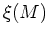is the initial mass function, andis the star formation rate; this implicitly assumes an IMF which is constant in time, which may or may not be true, but so far, there's no strong observational evidence against it (but note pop III issues).

• For any particular star, we use stellar evolution to compute amount and composition of mass returned to the ISM vs. amount locked up in stellar remnant ( Pagel Figure 2, from Maeder 1992). Note that the rate of return depends on stellar mass, especially for the case of SnIa, which also depends on the binary fraction.

• nucleosynthesis: main element groups and their sources:
• light elements: BBN
•elements: massive stars (core collapse SN)
• Fe-peak elements: type Ia SN and core collapse SN
• s and r process (neutron capture) elements: core collapse SN, AGB stars...

• Note that infall might arise from primordial clouds, or from processed material, e.g., mass-loss from halo stars. Outflow might come from supernovae winds, and in this case, it's possible that the composition of outflow material might be more enriched than the typical composition at any given time. So things can get complicated.

• Basic equations of chemical evolution

• Allowing for all sorts of realistic effects makes it difficult to make very simple predicions for the evolution of abundances. However, under some simplifying assumptions, it is possible to do so. A simple chemical evolution model serves as a useful baseline against which observations can be compared to determine where the assumptions may break down. In addition, understanding a simple model allows us to introduce and become acquainted with terminology that is is widespread usage.

• Consider a total mass M, split into a gas mass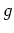and a total stellar mass,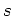. For a simple model, we'll consider that that gas at any time is well mixed.

At any given time, we wish to know the fractional abundance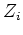of element. Consider an inflow rate into the system,, and outflow (ejection) rate,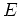. Then we have: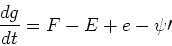whereis the star formation rate in units of mass per time,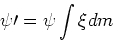and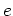is the ejection rate of mass from stars,which is a sum over all stellar masses of the product of the star formation rate at the time of formation of each mass with the mass returned to the ISM, weighted by the IMF. The lower limit of integration is at the stellar mass which is dying at time; lower mass stars don't contribute because they haven't ejected any mass yet. We also have,since the mass in stars increases by the number of stars formed, but decreases by the amount of mass lost back to the ISM from the previous generation of stars. For the elements, we havesince the mass in each element increases by the amount released by previous generations and added by inflow, but decreases by the amount locked up in new stars and lost to outflow. The termis given by:where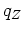represents the fractional mass of element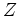synthesized and ejected during stellar evolution (so left term in brackets gives material which returns unprocessed and right term gives newly synthesized contribution). In a simple model, the synthesized masses are independent of the metallicity of the population (although we know this is not true for some elements, more on this later).

• To simplify, consider an approximation to these formulae called the instantaneous recycling approximation which assumes that all elements are returned instantaneously - good for products of massive stars, but less good for products from lower mass stars, e.g., iron. Then we havewhereis the lock-up fraction of mass locked up in remnants:where the second term is the total mass in stars which have died, and the third term is the mass from those stars which has been locked up in remnants. Alternatively, one can think of the return fraction,. This gives usWe also haveWe define the stellar yield,, to beso the yield give the fraction of the remnant population synthesized and released in each element. This gives us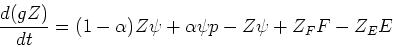where rhs terms are recyled material, new production, lock-up in new stars, inflow, and outflow. Simplifying, we have:Using mass locked up in stars,, as the independent variable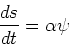so we getIf we make a further assumption of a uniform wind,, then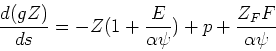and, finally,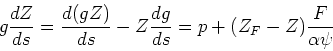or, alternatively,This is a basic equation of chemical synthesis.

• The simplest model for chemical evolution assumes no inflow or outflow, a homogeneous system without any spatial differentiaion of metallicity, zero initial metallicity, and yields which are independent of composition. This is known as the Simple, one-zone model.

In the Simple model, we haveSolving for Z(g), we getwhereis the gas fraction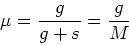The average abundance in stars is: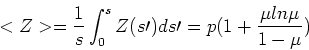which tends toasbecomes small, i.e., the gas fraction becomes small. For larger gas fractions, the meanwill be lower than the yield. Note that this is the mean metallicity weighted by stellar mass, not by stellar luminosity or by number, but these could easily be derived.

• Relative abundances of different elements
• Brief digression into units people use for measuring abundances:,,,.

• The chemical evolution relation holds for any element, so the ratio of the abundance of any two elements should be the ratio of the yields and constant in time. This seems to be true for some elements, e.g. Ne and S ( Pagel Fig 5, Pagel Fig 6), but not for others, e.g. C ( Fig 7). This is likely caused by a changing yield of carbon with metallicity because, e.g., of mass loss.

• However, this relation doesn't seem to hold for Fe ( Fig 9), which is plausibly explained by a significant fraction of Fe coming from SnIa, which have a time delay and so the simple model with instantaneous recyling doesn't hold. This is important to realize, because many direct metallicity'' determinations are made from Fe lines. If SnIa is the right explanation, one would expect a constant ratio of Fe/O for systems in which star formation proceeded rapidly (i.e., finished before SnIa go off), but in systems with more extended star formation, Fe/O would increase at higher O abundance. ( Pagel Fig 10). Different/Fe ratios are indeed found in populations that are thought to have formed over a short period of time (e.g. globular clusters).

• Most elements go in lockstep with O ( Fig 11).

• The constancy of element abundance ratio holds for primary elements, i.e. those that are produced from primordial abundance. In addition, some elements are secondary, which means that some element must previously exist in order for the secondary element to be created. An example is N, which is produced during the CNO cycle in greater abundance with a larger initial abundance of C. This is observed ( Fig 12).

• Abundances as function of gas fraction

Another prediction of the simple model is that abundances should increase as gas fraction decreases, with the slope of the relation giving the yield. A relation with the correct trend is observed in a sample of irregular and blue compact galaxies ( Fig 13), but the derived yield is much lower than predicted from stellar evolution; this may arise from lower yields at low metallicity or from preferential mass loss of processed material (e.g., from supernovae) in these low mass systems. If we consider the luminosity of a system to be related to the gas fraction, we might expect a relation between luminosity and abundance, which is in fact observed (fig 14).

Sometime people talk about the effective yield'' of a system, which is the yield derived from application of a simple model to data.

If we have the gas fraction decreasing as one goes towards the center of spiral galaxies, we expect to observe a metallicity gradient in the sense of the observed one. Note that the gradient in stars, as measured by the mean metallicity, is significantly shallower than that in gas.

• Abundances as function of time

The simple model predicts an increase of the metallicity with time. The rate of increase depends on the rate of star formation. If we assume that the SFR is proportional to the gas mass,, witha constant, then we getand we expect the abundance to increase linearly with time. This trend is roughly observed (Pagel Fig 17) in MW disk stars, albeit with large scatter.

• Number of stars as a function of metallicity

The simple model can be used to predict the number of stars expected as a function of metallicity. Rewriting our basic equationDifferentiating with respect to Z, we ge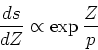which is a prediction for number of stars as a function of logarithmic abundance. This relation seems to work pretty well for field stars in the Galactic halo ( Fig 19), but only with a very low yield. This can be explained if one includes continuous mass loss from the halo system with a mass loss rate which is proportional to the star formation rate. Plausibly, this gas would flow into a disk and form disk stars. However, there's almost certainly not enough mass coming from the halo to form the entire disk. The metallicity distribution in the bulge also appears to fit a simple model reasonably well (Fig 22).

However, the distribution of disk field stars does not fit the simple model well at all. The problem is that there are too few observed low metallicity stars, and is commonly known as the G-dwarf problem (Fig 23). There are several possible solutions: time-dependent yields, a finite initial enrichment (or prompt initial enrichment'' from gas inflowing from the bulge or halo or a different initial IMF), or, probably most popular, inflow models. (fig 23 shows several inflow models).

• summary

General applicability, or lack theeof, of simple chemical evolution models. Utility of chemistry for fingerprinting'' populations.Next: Stellar populations and chemical Up: AY616 class notes Previous: Stellar populations
Jon Holtzman 2007-05-04